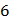### Sample Problem

Multiply mixed numbers with cancellation shortcut.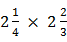=

#### Solution=Cancel the 4 and the 8 by dividing 4 into both of them: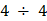==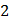. Cross out the 4 and the 8.Cancel the 9 and the 3 by dividing 3 into both of them: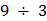==. Cross out the 9 and the 3.Multiply across the new top numbers and the new bottom numbers: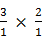==